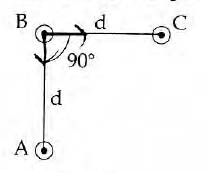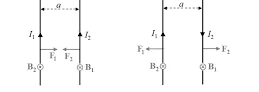#### An arrangement of three parallel straight wires placed perpendicular to plane of paper carrying same current 'I' along the same direction is shown in Fig. Magnitude of force per unit length on the middle wire ' B' is given by :Option 1)Option 2)Option 3)Option 4)As discussed in

Force between two parallel current carrying conductors -- whereinI1 and I2 current carrying two parallel wires

a-seperation between two wires

Force between wires A and B = Force between B and C

BC =net force on wire BOption 1)This solution is incorrect.

Option 2)This solution is incorrect.

Option 3)This solution is incorrect.

Option 4)This solution is correct.

#### Vakul